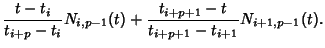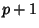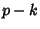## B-SplineA generalization of the Bézier Curve. Let a vector known as the Knot Vector be defined(1)

whereis a nondecreasing Sequence with, and define control points, ...,. Define the degree as(2)

The knots'', ...,are called Internal Knots.

Define the basis functions as(3)(4)

Then the curve defined by(5)

is a B-spline. Specific types include the nonperiodic B-spline (firstknots equal 0 and lastequal to 1) and uniform B-spline (Internal Knots are equally spaced). A B-Spline with no Internal Knots is a Bézier Curve.

The degree of a B-spline is independent of the number of control points, so a low order can always be maintained for purposes of numerical stability. Also, a curve istimes differentiable at a point whereduplicate knot values occur. The knot values determine the extent of the control of the control points.